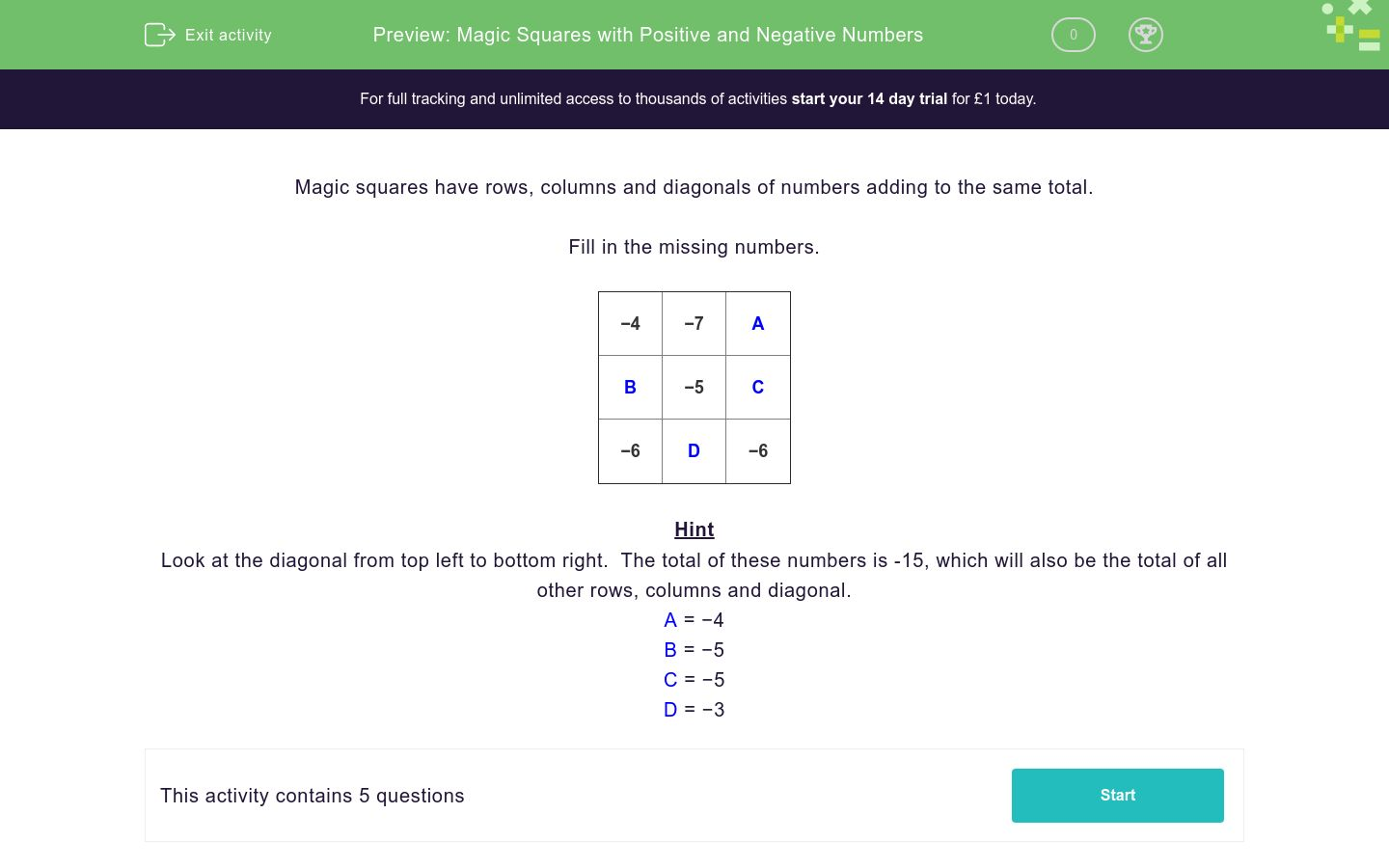# Magic Squares with Positive and Negative Numbers

In this worksheet, students solve problems with positive and negative numbers using 'Magic Squares'.Key stage:  KS 3

Curriculum topic:   Number

Curriculum subtopic:   Use Four Operations for All Numbers

Difficulty level:### QUESTION 1 of 10

Magic squares have rows, columns and diagonals of numbers adding to the same total.

Fill in the missing numbers.

 −4 −7 A B −5 C −6 D −6

Hint

Look at the diagonal from top left to bottom right.  The total of these numbers is -15, which will also be the total of all other rows, columns and diagonal.

A = −4
B = −5
C = −5
D = −3

Magic squares have rows, columns and diagonals of numbers adding to the same total.

Find the missing numbers.

 3 2 A 0 B 4 3 C D
 A B C D 1 2 3 4

Magic squares have rows, columns and diagonals of numbers adding to the same total.

Find the missing numbers.

 −4 −7 A B −5 C −6 D −6
 A B C D -5 -3 -4 -1

Magic squares have rows, columns and diagonals of numbers adding to the same total.

Find the missing numbers.

 5 A B 0 3 6 4 C D
 A B C D 1 2 3 4

Magic squares have rows, columns and diagonals of numbers adding to the same total.

Find the missing numbers.

 A 2 1 0 B 4 C 2 D
 A B C D 1 2 3 4

Magic squares have rows, columns and diagonals of numbers adding to the same total.

Find the missing numbers.

 −3 A −2 B −2 C D E F
 A B C D E F -1 -2 -3 -4
• Question 1

Magic squares have rows, columns and diagonals of numbers adding to the same total.

Find the missing numbers.

 3 2 A 0 B 4 3 C D
 A B C D 1 2 3 4
• Question 2

Magic squares have rows, columns and diagonals of numbers adding to the same total.

Find the missing numbers.

 −4 −7 A B −5 C −6 D −6
 A B C D -5 -3 -4 -1
• Question 3

Magic squares have rows, columns and diagonals of numbers adding to the same total.

Find the missing numbers.

 5 A B 0 3 6 4 C D
 A B C D 1 2 3 4
• Question 4

Magic squares have rows, columns and diagonals of numbers adding to the same total.

Find the missing numbers.

 A 2 1 0 B 4 C 2 D
 A B C D 1 2 3 4
• Question 5

Magic squares have rows, columns and diagonals of numbers adding to the same total.

Find the missing numbers.

 −3 A −2 B −2 C D E F